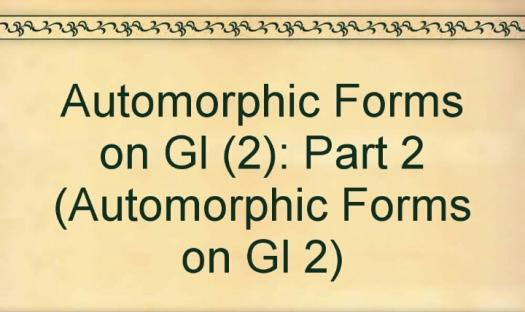# What Do You Know About Automorphic Forms On Gl(2)?

Approved & Edited by ProProfs Editorial Team
The editorial team at ProProfs Quizzes consists of a select group of subject experts, trivia writers, and quiz masters who have authored over 10,000 quizzes taken by more than 100 million users. This team includes our in-house seasoned quiz moderators and subject matter experts. Our editorial experts, spread across the world, are rigorously trained using our comprehensive guidelines to ensure that you receive the highest quality quizzes.
A
Community Contributor
Quizzes Created: 145 | Total Attempts: 38,511
Questions: 10 | Attempts: 114SettingsIn the 1970s, a French-American mathematician and an American-Canadian mathematician wrote, authored, and published the book Automorphic Forms on GL(2).
As seen in the book, the two mathematicians rewrote a certain theory of modular forms with respect to the adele rings of global fields and representation theory of GL(2) over local fields.

• 1.

### Who wrote the book Automorphic Forms on GL(2)?

• A.

H. Jacquet and Robert Langlands

• B.

H. Jacquet and Austin Langlands

• C.

H. Jacquet and Joel Langlands

• D.

H. Jacquet and Robert Langlan

A. H. Jacquet and Robert Langlands
Explanation
H. Jacquet and Robert Langlands wrote the book Automorphic Forms on GL(2).

Rate this question:

• 2.

### In which year was the book released?

• A.

1968

• B.

1969

• C.

1970

• D.

1971

C. 1970
Explanation
The book was released in 1970.

Rate this question:

• 3.

### Which theory was rewrote in the book?

• A.

Hecke's theory

• B.

Henry's theory

• C.

Charles's theory

• D.

Fibonacci's theory

A. Hecke's theory
Explanation
The correct answer is Hecke's theory.

Rate this question:

• 4.

### Which field was considered in the adele ring?

• A.

Local field

• B.

External field

• C.

Secured field

• D.

Global field

D. Global field
Explanation
The question is asking about the field that was considered in the adele ring. The correct answer is "Global field." This suggests that the adele ring, which is a mathematical concept in number theory, is associated with a global field. A global field is a type of field that includes both finite extensions of the rational numbers and the field of rational numbers itself. Therefore, the adele ring is related to the global field.

Rate this question:

• 5.

### Before beginning the study of automorphic forms, what representation theory are we going to review?

• A.

General linear groups

• B.

Global fields

• C.

Exponential functions

• D.

Linear equations

A. General linear groups
Explanation
Before studying automorphic forms, it is necessary to review the representation theory of general linear groups. This is because automorphic forms are functions on certain groups, and understanding the representation theory of these groups is crucial in analyzing and studying automorphic forms. The representation theory of general linear groups deals with the study of how these groups can be represented by matrices, and it provides the tools and techniques to analyze the behavior of automorphic forms on these groups.

Rate this question:

• 6.

### In weils representation, F = ?&nbsp; In Weils representation, what is F equal to?

• A.

Global field

• B.

Local field

• C.

Zeroth function

• D.

Geometric function

B. Local field
Explanation
In Weil's representation, F refers to a local field. A local field is a field that is complete with respect to a non-Archimedean absolute value. It is a field equipped with a norm that satisfies the triangle inequality and takes values in the non-negative real numbers. Examples of local fields include p-adic fields and the field of formal Laurent series.

Rate this question:

• 7.

### In Weils representation, K is equal to which of these?

• A.

Linear equations

• B.

Algebraic equations

• C.

Trigonometric functions

• D.

Exponential functions

B. Algebraic equations
Explanation
In Weil's representation, K is equal to algebraic equations. This means that K represents a set of equations that involve variables and constants, and can be solved using algebraic methods such as factoring, substitution, or the quadratic formula. This is different from linear equations, which involve variables raised to the power of 1, and from trigonometric and exponential functions, which involve variables raised to the power of angles or exponents, respectively.

Rate this question:

• 8.

### What do we use for representation in non-Archimedean cases?

• A.

GL(2,0)

• B.

GL(2,F)

• C.

GL(F,2)

• D.

GL(0,2)

B. GL(2,F)
Explanation
GL(2,F) is the correct answer because in non-Archimedean cases, GL(2,F) is used for representation. The notation GL(2,F) represents the general linear group of 2x2 matrices over the field F. This group is commonly used in mathematics to study linear transformations and their properties. Therefore, GL(2,F) is the appropriate choice for representation in non-Archimedean cases.

Rate this question:

• 9.

### For lemma 2.5, what does V1 represent?

• A.

Invariant subspace of V

• B.

Variant subspace of V

• C.

First order of V

• D.

Vector V

A. Invariant subspace of V
Explanation
V1 represents an invariant subspace of V. An invariant subspace is a subspace of a vector space that remains unchanged under the action of a linear operator. In this context, V1 is a subspace of V that is preserved by some linear transformation or operator.

Rate this question:

• 10.

### When was the second volume of the book published?

• A.

1969

• B.

1970

• C.

1971

• D.

1972

D. 1972
Explanation
The second volume of the book was published in 1972.

Rate this question:

Related TopicsBack to top